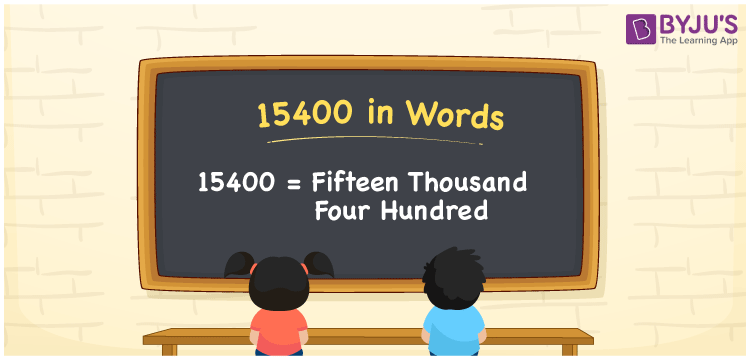# 15400 in Words

15400 in words is written as Fifteen Thousand Four Hundred. We can derive the word form of the number 15400 with the help of a place value chart. However, we use the word form of 15400 to express the value to others or convey something involving the use of the number 15400. Suppose to read the number 15400; we spell it as Fifteen Thousand Four Hundred.

 15400 in words Fifteen Thousand Four Hundred Fifteen Thousand Four Hundred in Numbers 15400

## 15400 in English Words

Generally, we use the English alphabet to write numbers in words. Therefore, we can read 15400 in English words as “Fifteen Thousand Four Hundred”.## How to Write 15400 in Words?

The number 15400 is a five-digit number, so we need to create a five-column place value chart to convert the number 15400 into words.

 Ten thousands Thousands Hundreds Tens Ones 1 5 4 0 0

In the above table,

ones = 0, tens = 0, hundreds = 4, thousands = 5, ten thousands = 1

By expanding the digits based on the place values, we get;

1 × Ten thousand + 5 × Thousand + 4 × Hundred + 0 × Ten + 0 × One

= 1 × 10000 + 5 × 1000 + 4 × 100 + 0 × 10 + 0 × 1

= 10000 + 5000 + 400

= Ten Thousand + Five thousand + Four hundred

= Fifteen thousand + Four hundred

= Fifteen Thousand Four Hundred

15400 is a natural number that is the successor of 15399 and predecessor of 15401.

15400 in words – Fifteen Thousand Four Hundred

Is 15400 an odd number? – No

Is 15400 an even number? – Yes

Is 15400 a prime number? – No

Is 15400 a composite number? – Yes

Is 15400 a perfect square number? – No

Is 15400 a perfect cube number? – No

## Frequently Asked Questions (FAQs) on 15400 in Words

Q1

### How do you spell 15400?

Using English words, we spell 15400 as Fifteen Thousand Four Hundred.
Q2

### How do you write Rs. 15400 in words on a cheque?

On a cheque, Rs. 15400 in words can be written as “Fifteen thousand four hundred rupees only”.
Q3

### How do you convert 15400 in words?

We can convert 15400 into words as: 15400 = 15000 + 400 = Fifteen thousand + four hundred = Fifteen Thousand Four Hundred.
Q4

### Is 15,400 divisible by 5?

Yes. 15,400 is divisible by 5. Any number which ends with 0 or 5 is divisible by 5.
Q5

### What is the value of 15,450 minus 50?

15,450 minus 50 will be 15,400 or 15,450 – 50 = 15,400